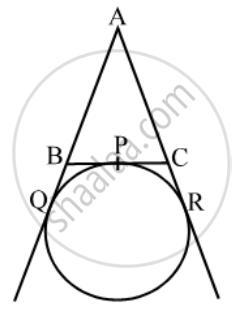Share

Books Shortlist

A circle touches the side BC of a ∆ABC at P, and touches AB and AC produced at Q and R respectively, as shown in the figure. Show that AQ = 1/2 - CBSE Class 10 - Mathematics

ConceptCircles Examples and Solutions

Question

A circle touches the side BC of a ∆ABC at P, and touches AB and AC produced at Q and R respectively, as shown in the figure. Show that AQ = \frac { 1 }{ 2 }

Solution

We know that the lengths of tangents drawn from an exterior point to a circle are equal.∴ AQ = AR …. (i) [tangents from A]

BP = BQ …. (ii) [tangents from B]

CP = CR …. (iii) [tangents from C]

Perimeter of ∆ABC

= AB + BC + AC

= AB + BP + CP + AC

= AB + BQ + CR + AC [using (ii) and (iii)]

= AQ + AR

= 2AQ [using (i)].

Hence, AQ = \frac { 1 }{ 2 }

Is there an error in this question or solution?

Video TutorialsVIEW ALL 

Solution A circle touches the side BC of a ∆ABC at P, and touches AB and AC produced at Q and R respectively, as shown in the figure. Show that AQ = 1/2 Concept: Circles Examples and Solutions.
S